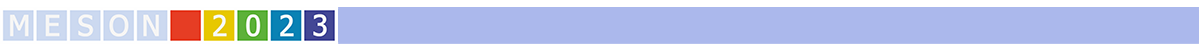#MESON2023

Jun 22 – 27, 2023
Auditorium Maximum
Europe/Warsaw timezone

## Derivative expansions of hadronic potentials coupled to quarks for X(3872)

Jun 22, 2023, 4:00 PM
20m
Medium lecture hall (A) (Auditorium Maximum)

Parallel

### Speaker

Ibuki Terashima (Tokyo Metropolitan University)

### Description

The inter-quark potentials diverge at large distance because of the color confinement of quarks. The inter-hadron potentials, on the other hand, vanish at large distance, because the interaction range is limited by the inverse pion mass. What then is the effect of the coupling to the inter-hadron potentials in the inter-quark potentials and vice versa?

We consider, in this talk, the channel coupling between the inter-quark and inter-hadron potentials. We then derive the effective potential of hadrons by eliminating the quark channel in the Feshbach method. In the case of the Yukawa type transition potential, we obtain the effective potential of hadrons,
\begin{align}
V(\mathbf{r},\mathbf{r'},E)
&= \frac{g_0^2}{E-E_0}\frac{e^{-\mu r}}{r}\frac{e^{-\mu r'}}{r'}, \tag{1}
\end{align}
where $g_0$ is the coupling constant of the channel transition between quarks and hadrons, $E_0$ is the energy of the bound state of the quark degrees of freedom. We show that the effective potential has non-locality and depends on the energy $E$.

There are two methods of converting non-local potentials into local ones. A formal derivative expansion decomposes directly a non-local potential in terms of the derivatives of the local potential. In the HAL QCD method, one derives the local potential from the wave function $\psi_{k_\mathrm{pot}}(r)$ at a momentum $k_\mathrm{pot}=\sqrt{2mE_\mathrm{pot}}$ which is obtained by the non-local potential.In the case of potential (1), we obtain at leading order,
\begin{align}
V^{\rm{{formal}}}(r,{E}) &= \frac{4\pi g_0^2}{\mu^2(E-E_0)}\frac{e^{-\mu r}}{r}. \tag{2}
\end{align}
\begin{align}
V^\mathrm{{HAL}}(r,E_\mathrm{pot}) &= E_\mathrm{pot}+\frac{-k_\mathrm{pot}^2\sin(k_\mathrm{pot} r+\delta)-\mu^2\sin\delta e^{-\mu r}}{2m(\sin(k_\mathrm{pot} r+\delta)-\sin\delta e^{-\mu r})}.\tag{3}
\end{align}

Finally, we take $c\bar{c}$ as the quark channel and $D\bar{D}^*$ as the hadron channel to apply this study to $X(3872)$. We construct the effective local $D\bar{D}^*$ potentials which reproduce the observed mass of $X(3872)$. By evaluating these local potentials numerically, we discuss the quantitative difference of local potentials in two methods. To study the effect of the local approximations in observables, we calculate the phase shifts from local potentials in comparison with the exact phase shift from the original non-local potential. In addition, we show that the phase shift is sensitive to the choice of $E_\mathrm{pot}$ in the HAL QCD method for the system with a weakly bound state such as X(3872).

 H. Feshbach, Ann. Phys. 5, 357 (1958); ibid., 19, 287 (1962).
I.Terashima and T.Hyodo, EPJ Web Conf. 271, 10004 (2022).
 S.Aoki and K.Yazaki, PTEP 2022, no.3, 033B04 (2022).
 A.Hosaka, T.Iijima, K.Miyabayashi, Y.Sakai, and S.Yasui, PTEP 2016 (2016).

### Primary author

Ibuki Terashima (Tokyo Metropolitan University)

### Co-author

Tetsuo Hyodo (Tokyo Metropolitan University)